# Conservation of Momentum

From Newton’s law, we know that the time rate change of the momentum of a particle is equal to the net force acting on the particle and is in the direction of that force.

$F_{net} = \frac{dp}{dt}$

This clearly makes us understand that linear momentum can be changed only by a net external force. If there is no net external force, p cannot change.

${{F}_{net}}=0;\frac{dp}{dt}=0\to p~is~constant$

Implies that there is no change in the momentum or the momentum is conserved. i.e

$initial \;momentum = final\;momentum$

In order to apply conservation of momentum, you have to choose the system in such a way that the net external force is zero.

Example:

In the example given below, the two cars of masses m1 and m2 are moving with velocities v1 and v2 respectively before the collision. And their velocities change to $v_{1}^{‘}~and~v_{2}^{‘}$ after collision. To apply the law of conservation of linear momentum, you cannot choose any one of the car as the system. If it so, then there is an external force on the car by another car. So we choose both the cars as our system of interest. This is why in all collisions, if both the colliding objects are considered as system, then linear momentum is always conserved (irrespective of type of collision).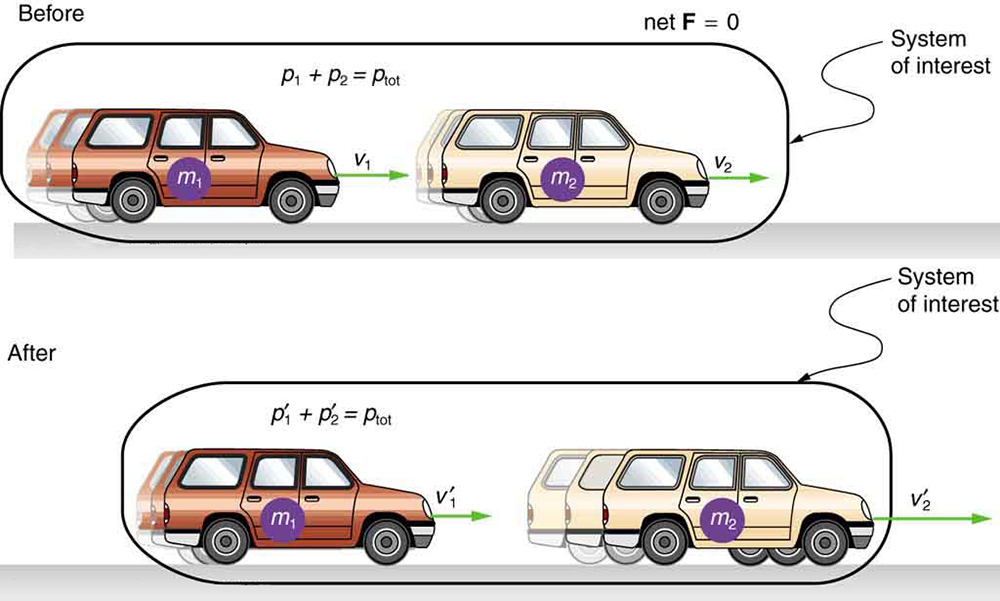Problem

A bullet of mass m leaves a gun of mass M kept on a smooth horizontal surface. If the speed of the bullet relative to the gun is v, the recoil speed of the gun will be_______________________.

No external force acts on the system (gun + bullet) during their impact (till the bullet leaves the gun). Therefore the momentum of the system remains constant. Before the impact (gun + bullet) was at rest. Hence its initial momentum is zero. Therefore just after the impact, its momentum of the system (gun+bullet will be zero)

$\Rightarrow M\vec{V}_b+M\vec{V}_g = 0$ $\Rightarrow m[\vec{V}_{bg}+\vec{V}_g]+M\vec{V}_g = 0$ $\vec{V}_g = -\frac{m\vec{V}_{bg}}{M+m}, where \left | \vec{V}_{bg} \right | = velocity\;of\;bullet\;relative\;to\;the\;gun = v$ $v_g = \frac{mv}{M+m}(opposite\;to\;the\;direction\;of\;v)$

## Types Of Collision

The common normal at the point of contact between the bodies is known as line of impact. If mass centers of the both the colliding bodies are located on the line of impact, the impact is called central impact and if mass centers of both or any one of the colliding bodies are not on the line of impact, the impact is called eccentric impact.

Direct impact/collision

If velocities vectors of the colliding bodies are directed along the line of impact, the impact is called a direct or head-on impact;

Example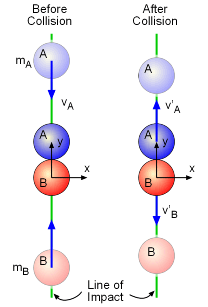Oblique impact/collision

If velocity vectors of both or of any one of the bodies are not along the line of impact, the impact is called an oblique impact.

Example

Consider a ball of mass m1 moving with velocity v1 collides with another ball of mass m2 approaching with velocity v2. After collision, they both move away from each other making an angle with the line of impact.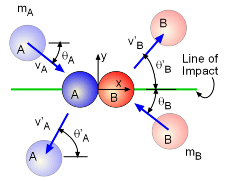Coefficient of restitution

When two objects collide head-on, their velocity of separation after impact is in constant ratio to their velocity of approach before impact.

$e = \frac{velocity\;of\;separation}{velocity\;of\;approch}$ $0\le e\le 1$ $velocity\;of\;separation = e(velocity\;of\;approach)$

The constant e is called as the coefficient of restitution.

### Elastic Collision

An elastic collision between two objects is one in which total kinetic energy (as well as total momentum) is the same before and after the collision.

Example

On a billiard board, a ball with velocity v collides with another ball at rest. Their velocities are exchanged as it is an elastic collision.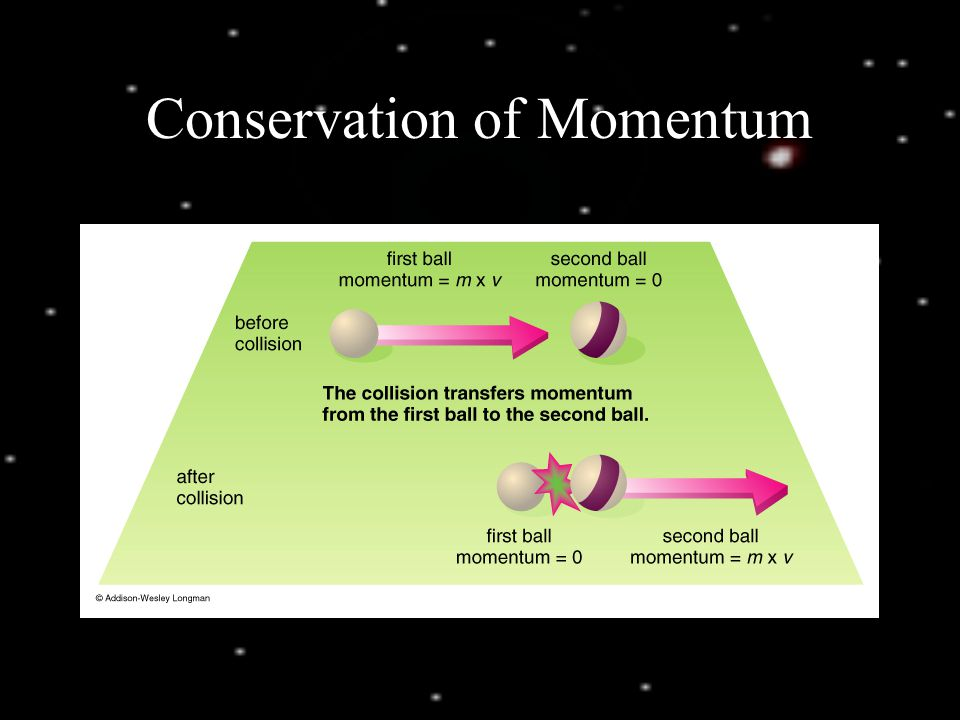### Inelastic Collision

An inelastic collision is one in which total kinetic energy is not the same before and after the collision (even though momentum is constant).

Examples

When the colliding objects stick together after the collision, as happens when a meteorite collides with the Earth, the collision is called perfectly inelastic.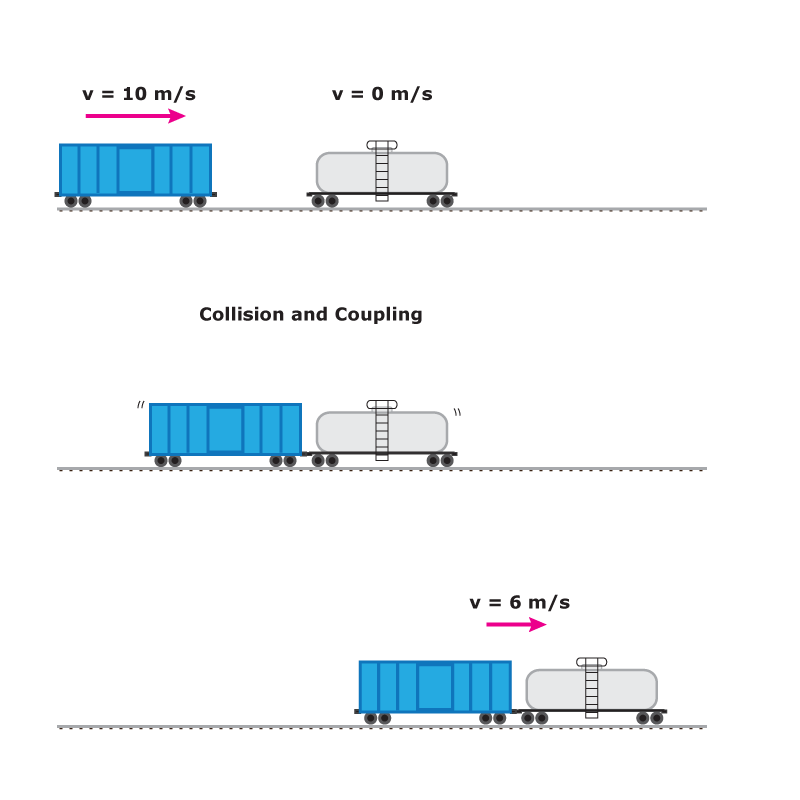In other inelastic collision, the velocities of the objects are reduced and they move away from each other.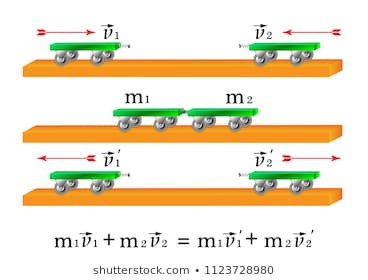### Velocities of colliding bodies after collision in 1- dimension

Let there be two bodies with masses m1 and m2 moving with velocity u1 and u2 . They collide at an instant and acquire velocities v1 and v2 after collision. Let the coefficient of restitution of the colliding bodies be e. Then, applying Newton’s experimental law and the law of conservation of momentum, we can find the value of velocities v1and v2.

Conserving momentum of the colliding bodies before and the after the collision

$m_1u_1+m_2u_2 = m_1v_1+m_2v_2$ ……(i)

Applying Newton’s experimental law

$We\;have\;\frac{v_2-v_1}{u_2-u_1}=-e$ $v_2 = v_1-e(u_2 – u_1)$…………………….(ii)

Putting (ii) in (i), we obtain

$m_1u_1+m_2u_2 =m_1v_1+ m_2{v_1-e(u_2 – u_1)}$ $v_1 = u_1\frac{(m_2-em_2)}{m_1+m_2}+u_2\frac{m_2(1+e)}{m_1+m_2}$……….(iii)

$From\;(ii)\;v_2 = v_1-e(u_2-u_1)$ $=u_1\frac{m_1(1+e)}{m_1+m_2}+u_2 \frac{(m_2-m_1e))}{m_1+m_2}$…………(iv)

When the collision is elastic; e = 1

$Finally,\;v_1 = \left ( \frac{m_1+m_2}{m_1+m_2} \right )u_1+\left ( \frac{2m_2}{m_1+m_2} \right )u_2$ $Finally,\;v_1 =\left ( \frac{2m_1}{m_1+m_2} \right )u_1+ \left ( \frac{m_2-m_1}{m_1+m_2} \right )u_2$

Having derived the velocities of colliding objects in 1 dimensional collision (elastic and inelastic), let us try to set conditions and draw useful conclusions.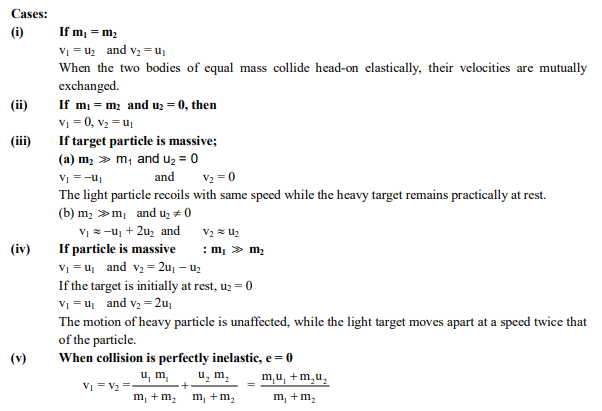### Elastic Collision in Two Dimension

A particle of mass m1 moving with velocity v1 along x-direction makes an elastic collision with another stationary particle of mass m2. After collision the particles move with different directions with different velocities.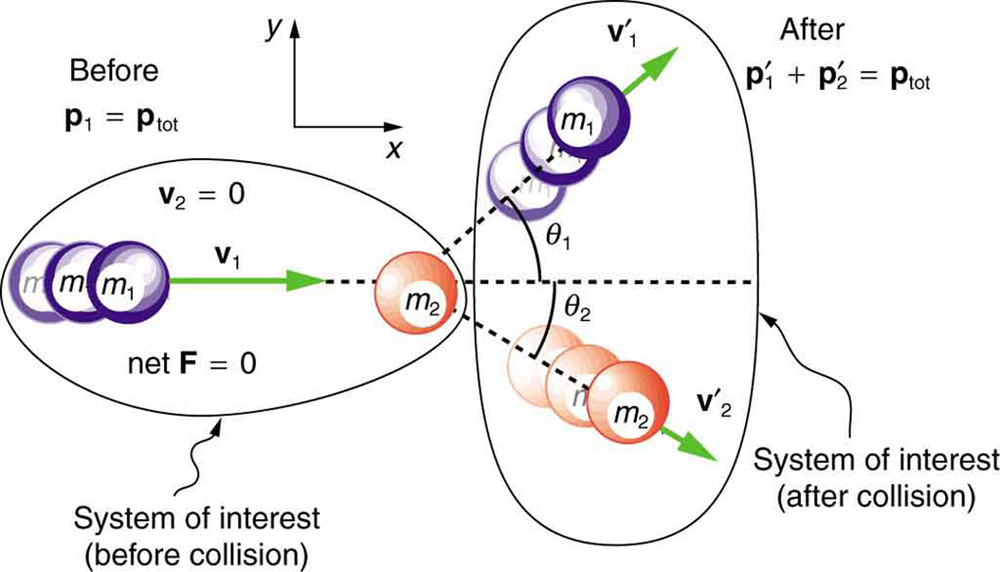Applying law of conservation of momentum,

Along x-axis:

$m_1V_1 = m_1{v_1}’\cos\theta_1+m_2{v_2}’\cos\theta_2$

Along y axis:

$0= m_1{v_1}’\sin\theta_1+m_2{v_2}’\sin\theta_2$

Conservation of kinetic energy,

$\frac{1}{2}m_1{v_1}^{2} = \frac{1}{2}m_1{{v_1}’}^{2}+\frac{1}{2}m_2{{v_2}’}^{2}$

Given the values of $\theta_1 and \;\theta_2$ , we can calculate the values of ${v_1}’ and \;{v_2}'$ by solving the above examples.

JEE Focus:

• Understand the conservation principle
• Make the right choice of system to apply the same

### Variable Mass System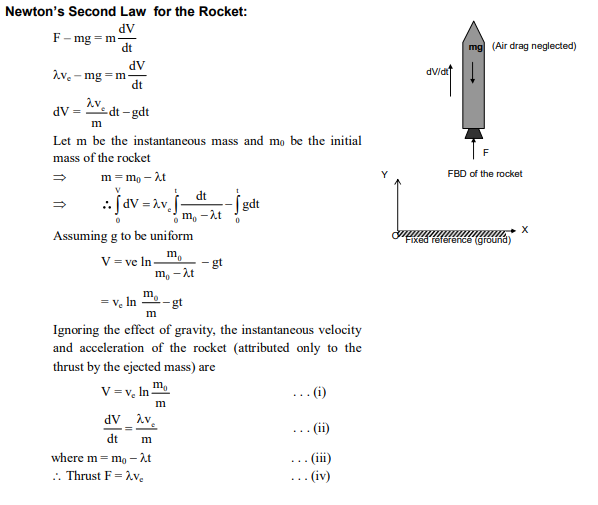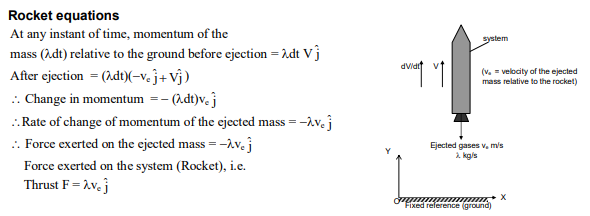Problem

After perfectly inelastic collision between two identical particles moving with same speed in different directions, the speed of the particles becomes (2/3)rd of the initial speed. The angle between the two particles before collision is

$(a)\cos^{-1}(-1/3)\;\;\;\;\;\;\;\;\;\;\;\;\;\;(b)\sin^{-1}(-1/3 )\;\;\;\;\;\; \\ \;\;\;\;\;\;\;\;(c)\cos^{-1(-1/9)}\;\;\;\;\;\;\;\;\;\;\;\;\;(d)\sin^{-1}(-1/9)$

Let $\theta$ be the desired angle. Linear momentum of the system will remain conserved. Hence

$P^{2} = P_{1}^{2}+P_{2}^{2}+2P_1P_2\cos\theta$ $=\left [ 2m\left ( \frac{2}{3} \right )v \right ]^{2}=(mv)^{2}+2(mv)^{2}+2(mv)(mv)\cos\theta$ $\Rightarrow \frac{16}{9} = 2+2\cos\theta$ $\Rightarrow 2\cos\theta= \frac{-2}{9}$ $\Rightarrow \cos\theta= \frac{-1}{9}$ $\Rightarrow \theta = 96.37^{0}$

Hence,(C) is the correct choice.